Digging Deeper into … Addition and Subtraction (infants to second class)

Digging Deeper into … Addition and Subtraction (infants to second class)

Category : Uncategorized

For practical suggestions for families, and links to useful digital resources, to support children learning about the topic of addition and subtraction, please check out the following post: Dear Family, your Operation Maths Guide to Addition and Subtraction

A quick look at the maths curriculum for junior and senior infants will reveal that, within the strand of number, there are no strand units entitled operations, addition or subtraction, as are evident in the curriculum for first and second classes. However both operations are there – under the guise of combining, partitioning and comparing.

Addition and subtraction are two of the four basic mathematical operations (multiplication and division being the other two):

• Addition involves the joining/combining of two or more quantities/sets/parts to get one quantity/whole/set, typically referred to as the sum or total. There are two main types: active (2 children at a table and 3 more join them) or static (2 boys and 3 girls at a table, how many children in all?)
• There are three types of subtraction:
• take-away (active) which involves the removal/deduction of one quantity/part from a whole amount/quantity
• comparison (static) which involves identifying by how much one quantity/set is more or less than another (the difference)
• missing addend (active) which involves identifying the amount needed to combine with a known part to make a whole.

In each type of subtraction we know the total/whole and a part and we need to find the missing part, which could be the amount left, the difference or the missing addend.

The types of addition and subtraction are explained very clearly and succinctly in the Origo One videos below.

As shown in the videos above, addition and subtraction are inverse operations; we can demonstrate addition by adding more to an existing amount; the reverse action would involve removing an amount, thus demonstrating subtraction as take away. In contrast to traditional maths schemes, which often have separate chapters for each of these operations, Operation Maths predominantly teaches addition and subtraction together, as related concepts. Teaching the operations in this way will encourage the children to begin to recognise the relationships between addition and subtraction.

Beginning in first and second classes, the children are enabled to understand addition and subtraction as being the inverse of each other, which will progress towards using the inverse operation to check calculations in higher classes.

CPA Approach within a context

As mentioned repeatedly in previous posts, both the Operation Maths and Number Facts series are based on a CPA approach. Furthermore, as was referenced in the videos above, for the children to develop a deep understanding of the different types of addition and subtraction, there has to be some context or story, with which they can identify. This, in turn, should be explored via progression through concrete, pictorial and abstract stages.

This context can be simply made up by the teacher or be inspired by a picture book that the class is reading. It can be modeled using the concrete materials available in the classroom (eg plastic animals, toy cars, play food etc. ) and/or using the Operation Maths Sorting eManipulative (see below) and the extensive suite of inbuilt images; the images can be shown either with or without a background (background options include five and ten frames, set outlines and various grids).HINT: To find out more about how to use the 5, 10 and 20 frames that accompany the Operation Maths series please read on here: http://operationmaths.ie/youve-been-framed-closer-look-ten-frames/

As the children progress, the need arises to record the operations using some graphic means. Initially, this can include representing each of the items in the story with counters and/or cubes. In turn, bar models could also be used to represent number relationships, while bearing in mind that different types of bar models will be required to model different context and types of subtraction (even though the subtraction sentences, if using them, might look exactly the same). Using the examples below, the first bar model (a part-whole bar model) could be used to model this story: Snow White had seven dwarfs. If four of these went to work, how many were left at home? Whereas, the second bar model (a comparison bar model) would better suit this story: the seven dwarfs all wanted to sit down at the table but there were only four chairs. How many dwarfs had no chair?While bar models do not specifically appear until in the pupils books until Operation Maths 3, the children could use and explore simple bar models. Thinking Blocks Jr is based on simple bar models and could be shown to the class on the IWB while the children suggest answers and labels on their Operation Maths MWBs.  Then the children could draw simple models in their books to help solve the word problems there. Furthermore , as shown above, the Bar Modelling eManipulative could also be used to create bars of different length.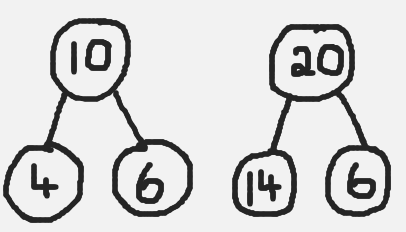Before rushing too quickly into abstract recording (using only digits and symbols), an alternative intermediary stage could be to represent the relationships, using a branching bond (opposite). Similar to the part-whole bar model earlier, this branching bond structure encourages the children to appreciate that two sets/parts ( 4 and 6) can be combined to make a larger set/whole (10). Inversely, when a part (4) is removed from the whole (10), a part is left (6). This bond structure can also represent the missing addend type of subtraction: if a part was hidden (6), the question could be asked  what must be added to 4 to make 10.

Both branching bonds and simple bar models are used throughout the Number Facts series to represent relationships and demonstrate strategies. They are also used throughout the Operation Maths 3-6 books, but in increasingly more complex situations.

The meaning of the equals sign

With the formal introduction of addition number sentences in senior infants (ie the recording of relationships using the plus and equals sign), followed by the formal introduction of subtraction sentences (using the minus sign) in first class, comes the need to correctly interpret the purpose of the equals sign as identifying equivalence; ie that the value on one side of the equals sign is the same as the value on the other side. It is essential at this stage that the children don’t interpret the equals signal incorrectly as being a signpost indicating that the answer is coming next. A pan or bucket balance is an extremely valuable resource to help demonstrate equivalency, as can be seen in the video below.

Calculations in the Operation Maths book are often shown vertically and horizontally. When presented horizontally, it is often misinterpreted that the children must now rewrite the calculation vertically, to be solved using the traditional column method (see more on the column method in the next section). Rather, presenting calculations horizontally is a deliberate effort to encourage the children to explore how to solve the calculation using a concrete based approach and/or using a mental strategy, as opposed to always tackling these calculations in a written way.

Looking at more complex numbers

In first and second classes, once introduced to operations using two-digit numbers, children can often have tunnel vision (or column vision) regarding addition and subtraction calculations: they “do” the units, and then the tens, without really looking at the whole numbers or the processes involved.

One way in which you can encourage the children to look at and understand these operations better is by using a CPA approach. This means that the children’s initial experiences should involve groupable base ten concrete materials (e.g. bundling straws or lollipop sticks, ten-frames and counters, unifix or multi-link cubes arranges in sticks of ten, see below), where a ten can be physically decomposed  into ten units and vice versa, before moving on to pregrouped base ten materials (eg base ten blocks/Dienes blocks, base ten money and/or Operation Maths place value discs) which require a swap to exchange a ten for ten units and vice versa.When children are comfortable with the manipulating the concrete materials, they can move on to a process whereby these materials are represented pictorially and/or demonstrate the process using a suitable the visual structure eg an empty number line and/or bar model. Abstract exercises, where the focus is primarily on numbers and/or digits, should only appear as part of the final stage of this process.When exchanging tens and units (or tens and hundreds in second class), reinforce that a ten is also the same as 10 units, and that a hundred is the same as 10 tens and is the same as 100 units. The use of non-canonical arrangements of numbers (e.g. representing 145 as 1H 3T 15U or  14T 5U), as mentioned in Place Value, can also be very useful to children as they develop their ability to visualise the regrouping/renaming process. The Operation Maths Place Value eManipulative, accessible on edcolearning.ie,  is an excellent way to illustrate this and explore the operations in a visual way.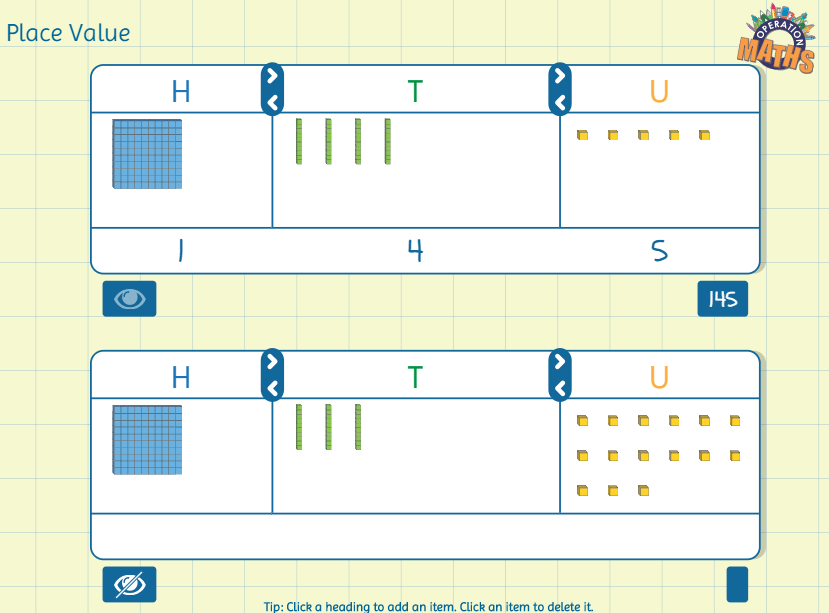Mental strategies are as important as written methods

In first and second classes, the traditional, written algorithms for addition and subtraction, i.e. the column methods, are important aspects of these operations. However, in real-life maths, mental calculations are often more relevant than written methods. Also, as mentioned previously, children can often have tunnel vision (or column vision) regarding addition and subtraction calculations; they ‘do’ the units, then the tens, without really looking at the entire numbers or the processes involved. Therefore, while the column method for addition and subtraction is an important aspect of this topic, equally important is the development of mental calculation skills, via a thinking strategies approach.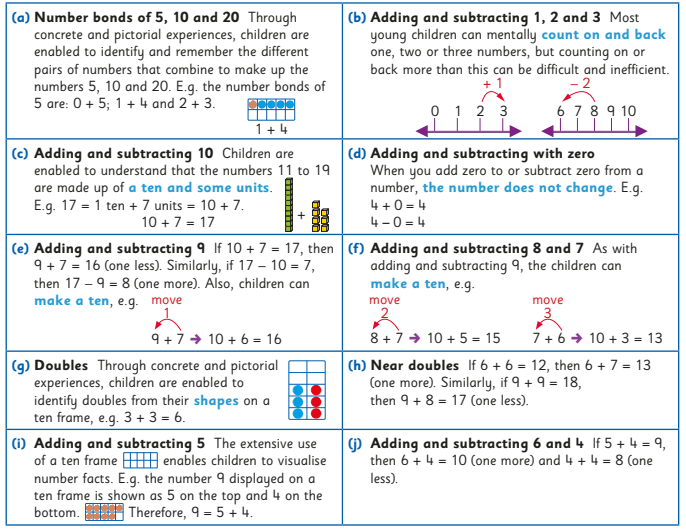From Number Facts 1 & 2

Thus, one of the main purposes of the operation chapters in Operation Maths is to extend the range of strategies that the children have and to enable them to apply the strategies to numbers of greater complexity i.e. for the children to become efficient and flexible, as well as accurate. As the same calculation can often be done mentally in many different ways, the children have to develop their decision-making skills so as to be in a position to decide what is the most efficient strategy to use in each situation.When meeting new calculations, ask the children, as often as possible, can they do it mentally, and how, so that they become increasingly aware of a range of mental calculation skills and approaches. In this way the children will also be developing their decision-making skills, so as to be in a position to decide the most efficient strategy/approach to use.

HINT: Number Talks are a fabulous resource to use alongside the Operation Maths and/or Number Facts series, as they complement their thinking strategies approach. Read on here to find out more about where both Operation Maths and Number Talks overlap.

Key messages:

• There are different types of addition and subtraction and children need to explore the different types to gain a deep understanding of the concepts
• As children encounter new numbers and new number ranges, be it numbers to ten in infants, teen numbers to 199 in first and second classes, they should be afforded ample opportunities to combine to make these amounts, partition these amounts and compare these amounts using concrete materials and via some story-like context.
• Initial recording of these relationships should be via counters and cubes etc, before moving on to pictorial representations of the same and/or using frames, maths rack, bar models, branching bonds etc.
• Addition and subtraction number sentences, that use only digits and symbols, should be avoided until the children demonstrate readiness for this more abstract stage.
• Encourage the children to use and develop mental strategies and avoid focussing almost exclusively on the formal, traditional ways of doing addition and subtraction ie column method.

This short video from Graham Fletcher showing the progression of addition and subtraction from the infant classes to the formal written algorithm, with three and four-digit numbers, is very worthwhile viewing and summarises the key messages well.

• Dear Family, your Operation Maths Guide to Addition and Subtraction includes practical suggestions for supporting children, and links to a huge suite of digital resources, organised according to class level.
• Operation Maths Digital Resources: As always don’t forget to access the linked digital activities on the digital version of the Pupil’s book, available on edcolearning.ie. Tip: look at the footer on the first page of each chapter in the pupil’s book to get a synopsis of what digital resources are available/suggested to use with that particular chapter.
• For more hints and tips specific to each class level, check out the “What to look out for” section in the introduction to this topic in the Teacher’s Resource Book (TRB)
• Number Talks book by Sherry Parrish
• Mental Maths handbook for Addition and Subtraction from the PDST
• Splat! Similar to Number Talks, these free resources from Steve Wyborney encourage discussion and reasoning. Play the PowerPoint presentations on your class IWB while the children use their Operation Maths MWBs to respond.
• Addition & Subtraction Board on Pinterest

Thinking Strategies for Addition and Subtraction Number Facts

Category : Uncategorized

What are number facts?

Number facts are the basic number facts that, it is hoped, children could recall instantly, so as to improve their ability to compute mentally and use written algorithms. Traditionally referred to as tables, the addition and subtraction number facts typically include all the addition facts up to 10 + 10 and their inverse subtraction sentences.

Some of the big ideas about number facts:

• Some facts are easier than others to recall – which ones, do you think?
• The easier facts can be used to calculate other facts – which ones, do you think?
• The same fact can be calculated using various approaches – these approaches are often referred to as thinking strategies – see more below.
• Using thinking strategies means that the children can apply the understanding, to facts beyond the traditional limits of “tables”.

What are thinking strategies?

A thinking strategy is a way to think about a process to arrive efficiently at an answer. For example, if asked to add 9 to a number, one could think of moving 1 from the other addend to the 9 so as to make a 10, which therefore becomes an easier calculation (see below)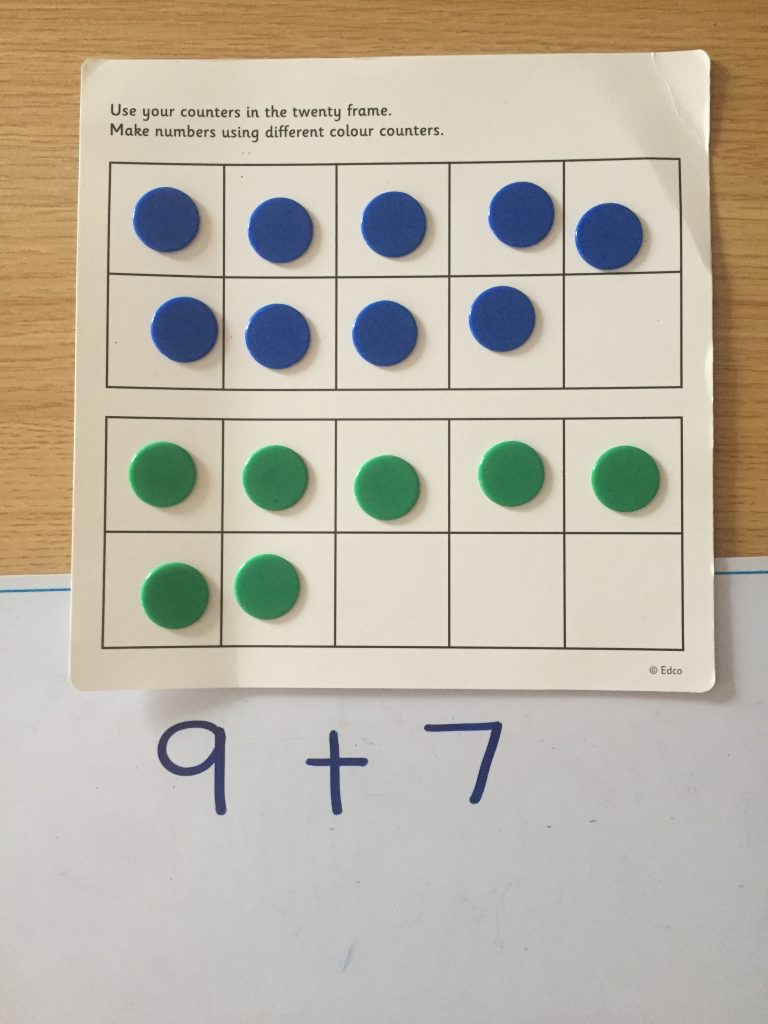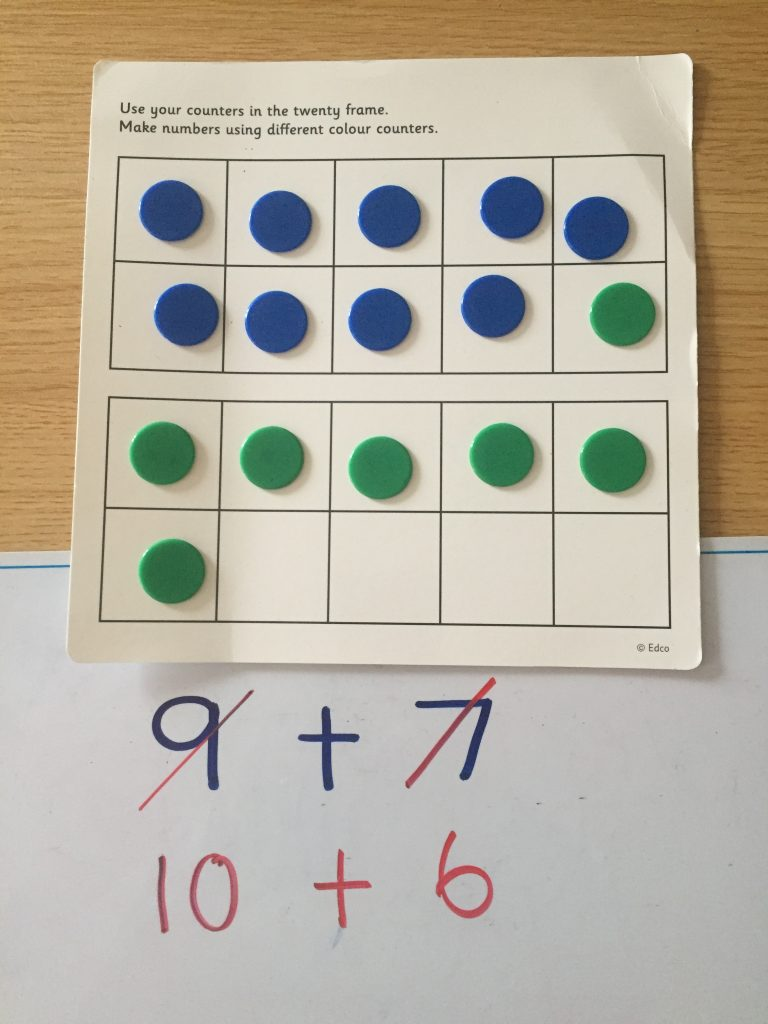The Operation Maths books for first and second classes emphasise three specific thinking strategies throughout: counting on from the biggest number, using doubles and near doubles and using the number bonds for ten (see image below). The doubles facts and bonds of ten are also included on the pull-out flap at the back cover to the pupils books, both for quick reference and to emphasise their importance.From Operation Maths 2 At School Book

In the case of doubles, near doubles and bonds of ten, these key sets of number facts tend to be easier for children to understand and recall. These facts also make up a core section of the total addition facts to 10 + 10, as highlighted below on the addition square. When these become known facts, they can then in turn be used to calculate unknown facts (eg if 7 + 3  = 10, then 7 + 4 = 11), thus covering an even greater number of the total addition facts.Furthermore, addition and subtraction are taught together throughout the Operation Maths series, so that, rather than compartmentalising each operation, the children develop a better understanding of how both concepts relate to each other. In this way, the basic subtraction facts are easier to acquire, as they are understood to be the inverse of the more familiar addition facts.

Traditionally, learning “tables” had been by rote, but current research suggests that this is ineffective for the majority of children. In contrast, children should be taught to visualise numbers and to use concrete materials, images and thinking strategies to use what they know to solve what they do not know. Below are examples of some useful thinking strategies for the basic addition and subtraction facts (taken from Number Facts 1 & 2, Edco, 2018)From Number Facts 1 & 2

There can often be different ways to think about the same fact (or groups of facts), and the children should always be encouraged both to identify alternative approaches and to choose their preferred strategy. For example:

8 + 6 = (5 + 3) + (5 + 1) = 10 + 4 (make a ten) = 14
8 + 6 = 10 + 4 (move 2 from 6 to 8 to make a ten) = 14
8 + 6 = 7 + 7 (move 1 from 8 to 6 to make a double) = 14

Once the children understand how to arrive at an answer via a thinking strategy, they can then apply this thinking strategy to more complex calculations that are beyond the traditional 10 + 10 ceiling of “tables”; for example if I understand different ways to calculate that 8 + 6 = 14, then I can use these ways to mentally calculate 18 + 6 , 18 + 16 etc.

Computational Fluency:

‘Fluency requires the children to be accurate, efficient and flexible.’ (Russell, 2000).

The primary aim of both the Operation Maths and Number Facts series (see more information on Number Facts below) is to enable the children to become computationally fluent. To achieve computational fluency, the children must be accurate, efficient and flexible:

• Accurate: the children must arrive at the correct answer, e.g. 8 + 6 = 14.
• Efficient: the children must calculate the answer in an efficiently. A child who produces an answer of 14 in response to the question 8 + 6 by ‘counting all’ (eg have to count up to a total using using counters, fingers, etc.) may be accurate but is not efficient.
• Flexible: children must be able to visualise and mentally manipulate numbers in order to see how they might be broken down and recombined to get an accurate and efficient answer (as shown with the various ways to consider 8 + 6 above).

Thus, flexibility is the key to fluency. A child who only knows that 8 + 6 = 14 becasue they have memorized that fact, is missing out on all the various possible connections between those numbers, subsequently hampering future connection-building. In contrast, a child who is flexible with number facts is one with a well-developed number sense, who can see the connections both between and within numbers, i.e. they can partition and/or combine numbers into more compatible (friendly) amounts and can apply their strategies to numbers beyond those they have dealt with. Thus, a thinking strategies approach will not only be effective for aiding understanding and recall of the basic facts up to 10 + 10, a thinking strategies approach can enable children to apply these mental computation skills to numbers beyond this traditional ceiling e.g. 19+ 5, 29 + 6 etc (see below).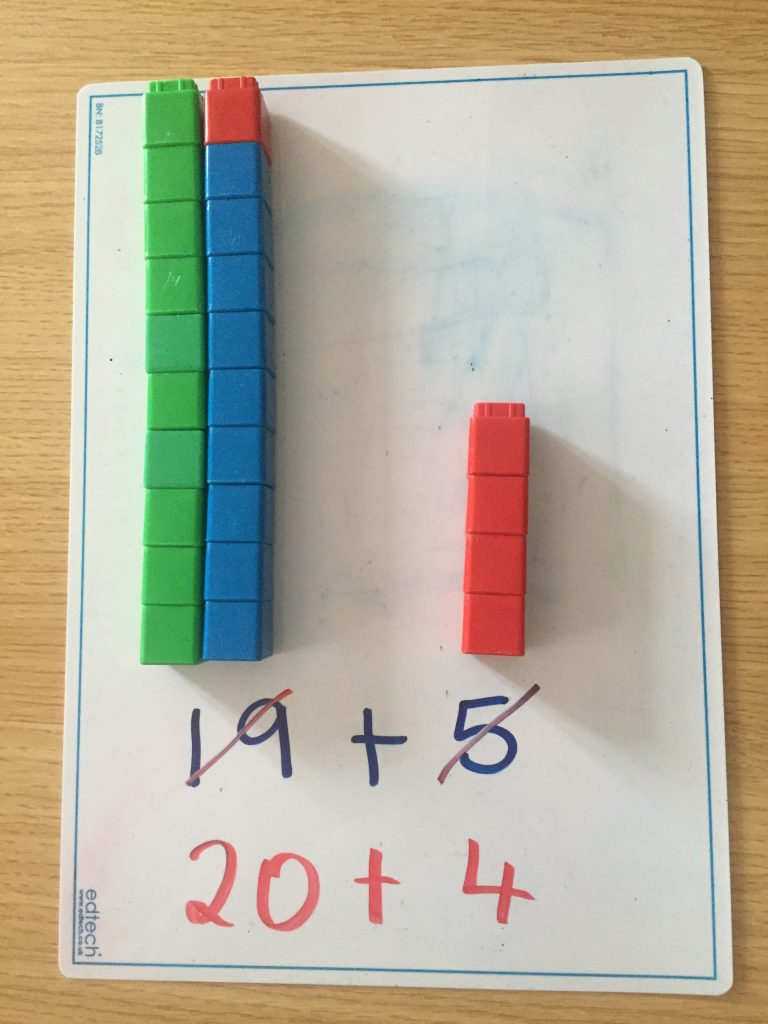The Number Facts Series from Edco

Number Facts is latest addition to the Edco Primary Maths stable, and it is a series of activity books designed to foster fluency in number facts for primary school children from First Class. The series features an innovative approach to the acquisition of basic number facts, and, like Operation Maths, teaches children to understand, not just do, maths.In contrast to the more traditional drill-and-practice workbooks, which just test whether the answer is known, Number Facts teaches children to visualise numbers pictorially and to use these images and thinking strategies to become more adept at manipulating numbers. The specific focus of Number Facts will be to develop children’s thinking strategies and apply these to the basic number facts in such a way as to promote the child’s ability to visualise and recall these facts, thereby achieving fluency.

Both this rationale, and the suggested teaching approaches to the teaching of the basic addition and subtractions facts for first and second classes, are clearly outlined in the Teachers Resource Book (TRB) which accompanies the series, and which is downloadable here. This TRB also includes a Long Term Plan for both first and second classes (see extract below), outlining a logical progression for the various fact groups throughout the school year. To view sample pages from the pupils Number Facts books please click here. Sample copies of all the books are also available from your local Edco reps.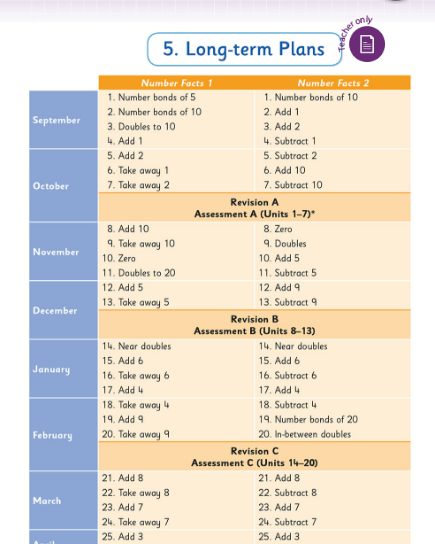• Are you compensating? A closer look at the thinking strategy of compensation.
• Number Talks : this is a maths methodology centered around the development of  strategies and mental calculation skills. As such, it really complements both the Operation Maths and Number Facts series. For more information on where Operation Maths and Number Talks overlap, please read on here.
• Mental Maths handbook for Addition and Subtraction from the PDST
• Number Facts Board on Pinterest
• The Origo One videos below are a great way to get an overview of some various thinking strategies, each in 60 seconds or less!

For practical suggestions for families, and links to useful digital resources, to support children learning about the topic of addition and subtraction, please check out the following post: Dear Family, your Operation Maths Guide to Addition and Subtraction

Digging Deeper into … Multiplication and Division (3rd – 6th classes)

Category : Uncategorized

For practical suggestions for families, and links to useful digital resources, to support children learning about the topic of multiplication and division, please check out the following post: Dear Family, your Operation Maths Guide to Multiplication and Division

Children are formally introduced to the operations of multiplication and division in third class, although their initial exploration of these concepts would have begun in 1st and 2nd classes with basic skip counting in jumps of 2s, 10s, 5s and, to a lesser extent, 3s and 4s. Thus, most children become quite comfortable with the number facts of twos, fives and tens quite quickly because these facts are more familiar to them. That said, when starting multiplication and division, and particularly when doing these groups of “easier” facts, it is still very important that the children are given ample time and experiences to develop a solid understanding of the concepts of multiplication and division themselves. This is why this topic is typically taught as a double (i.e. two week) chapter in Operation Maths.

Furthermore, as with the Operation Maths approach to addition and subtraction, multiplication and division is, for the most part, taught together, so as to reinforce them as related concepts that are also the inverse of each other. Thus, the initial activities in the Discovery Book, often require the children to reflect on their understanding of the concepts and to compare and contrast them.

As the children move through the classes, it is anticipated that they would begin to use this understanding of multiplication and division as the inverse of each other, to recognise the quotient (answer) in division as a missing factor in the inverse multiplication fact, e.g. asking what is 45 ÷ 9 is the same as asking how many groups of 9 equals 45 or [ ] × 9 = 45. This knowledge will also help as the children move towards more efficient recall of the basic division facts.

CPA Approach

As always, Operation Maths advocates a concrete–pictorial–abstract (CPA) approach to this topic. In 3rd class, this means the children will be moving from experiences with familiar objects that are already pregrouped (eg three wheels on a tricycle, four wheels on a car, ten toes on a person) and/or groups they create themselves using objects, to pictorial activities (e.g. where the children draw representations of the numbers using  pictures of the concrete materials) and finally to abstract exercises, where the focus is primarily on number sentences and the use of the multiplication and division symbols.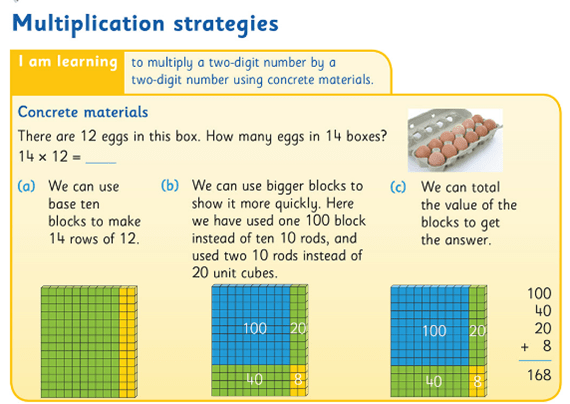Using base ten blocks to demonstrate multiplication as an area array

Similarly, in 4th to 6th classes, the approach taken should start with concrete materials (eg base-ten blocks to represent multiplication using the area model, as shown above) as demonstrated by the images in the Pupil’s books and explained in the relevant Teacher’s Resource Book (TRB). As always, the emphasis is on the children understanding the process before being introduced to the formal algorithms (abstract stage). Otherwise, they are just being taught to use a procedure to produce an answer without really understanding why or how it works.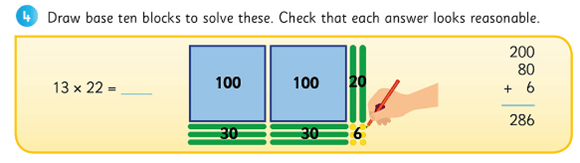Moving on from the actual blocks; drawing an area model as a pictorial representation of the calculation.

Teachers should use opportunities such as questioning, conferencing etc. to assess their children’s understanding as opposed to just checking for a correct answer.  In particular, check does the child truly understand the purpose of the zero in the second line of the long multiplication algorithm; often when questioned a child will say they need a zero or the answer will be wrong, without appreciating that they are now multiplying by a multiple of ten, so the digits must be moved up a place, hence the necessary zero as placeholder. Therefore, in the example below, 14 is first multiplied by 7, giving a product of 98 on the first line, and then 14 is multiplied by 10 (not 1) giving a product of 140 on the second line. These partial products are then added to give a final total or final product. If in doubt about a child’s understanding, return to the concrete materials and/or pictorial phase again.  Based again on the area model of multiplication, the Partial Product finder tool from the Math Learning Centre can also be very useful to demonstrate this (shown below).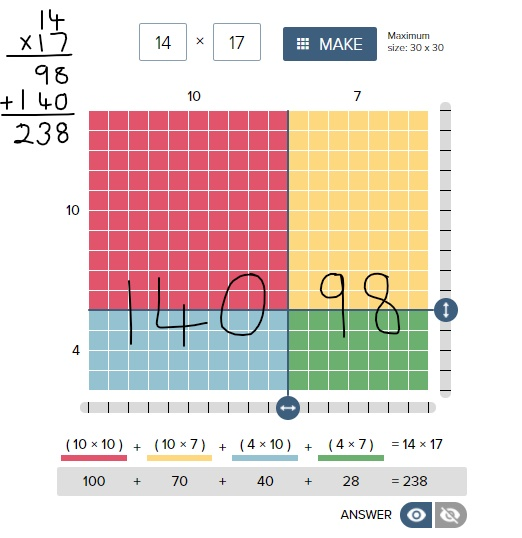If you want even more examples of the effectiveness of using the area model of multiplication, please check out this video from James Tanton. The entire facebook post is accessible here

Similarly, when considering division, while the goal is for the children to be able to divide by two-digit numbers confidently, that doesn’t necessarily have to be via the formal long division algorithm. The long division algorithm has long been a controversial element in primary mathematics. Many feel it is counter-productive to be spending so much time in 5th and 6th class learning and teaching a procedural approach to solve written calculations, when the children will have continual access to a calculator for such calculations once they hit secondary school. In fact, in many other educational systems (UK and various US states included) they have abandoned the teaching of the long division algorithm completely in preference to the ‘chunking method’ (also known as the partial quotients method). However, if the method of the long division algorithm is taught using a CPA approach, as demonstrated in the Operation Maths Pupil’s book for 5th class, it can serve to enhance children’s understanding of number and operations in general.Language & PropertiesConsistent and accurate use of language is also an important way to emphasise the necessity of this interconnected CPA approach. The way that the number sentences for these operations are verbalised can unwittingly confuse children. If for  2 x 4, we say ‘two multiplied by four’, that implies 2 + 2 + 2 + 2, e.g. four plates with two cookies oneach. However, if we say ‘two times/groups/rows of four’, that implies 4 + 4, e.g. two plates with four cookies on each. While it is understood that the order for multiplication doesn’t matter (i.e. the commutative property of multiplication) and that the answer for both of these is the same, children don’t inherently understand this, especially at the introductory stage. And to further confuse them, when represented concretely or pictorially, both images would also look different.

Therefore, for consistency, initially teachers and students  should use  the language of ‘groups/rows of’ to describe multiplication sentences and use the word times when verbalising the multiplication symbol (×). Similarly, in division we should initially use ‘shared/divided between’ and when using the division symbol (÷) we should say ‘divided into (… equal groups)’. When comfortable with the concept of division as sharing (i.e. 12 ÷ 2 = 6, 12 divided into 2 equal groups is 6 in each group) then the children can be introduced to the concept of division as repeated subtraction (12 ÷ 2 = 6, how many groups of 2 in 12 or how many times can I take  a group of 2 away from 12).

Once the children understand that they can use the commutative property to turn around facts in order to calculate more efficiently, they should be encouraged to recognise how multiplication sentences can be distributed into smaller groups, and that smaller groups can be combined to give the same total i.e  the distributive property of multiplication. It is hoped that they will begin to recognise where these properties can be applied in order to make calculations easier. Encourage the children to read distributed number sentences using the language of ‘groups of’ or ‘rows of’, as this can aid clarity, where the numbers and symbols all together can cause confusion. The 100 dots grids on the inside back covers of Operation Maths 3 and 4 can be extremely useful for the pupils to model various arrangements/arrays, while the teacher can use the 100 square eManipulative to replicate the children’s arrangements on the IWB.Ultimately, being able to understand and apply the properties of multiplication greatly aids fact fluency. Understanding the distributive property, in particular, is key to understanding how the long multiplication algorithm works, i.e. that we multiply by the units and then multiply by the tens and then recombine these partial products. The distributive property and the ability to combine or break apart groups within factors also provides the children with strategies to mentally calculate products and quotients without having to use the standard written algorithms, for example using branching and T-charts, as featured in Operation Maths 5.

Thinking Strategies for the Basic Number Facts

When most of us were in school, multiplication and division was largely all about tables and learning off tables. Yet, research into this area in recent years suggests strongly that memorisation and rote learning is not an effective strategy; that even when successful (which may not happen for all), the children are learning off the basic number facts, without fully understanding them, and the connections both within and between these groups of facts. Therefore, while instant recall of the basic facts (up to 10 times and divided by 10) is a primary goal of both the mathematics curriculum and of Operation Maths, Operation Maths places significant emphasis on equipping the children with thinking strategies that will enable them recall the basic facts and to compute mentally, even with numbers that are outside the traditional limits of the tables.

Some of these strategies include utilising a child’s understanding of division as the inverse of multiplication, the properties of multiplication (e.g. identity, zero, commutative and distributive) which in themselves lead into exploring connections such as doubling and halving, thirding and trebling and facts as being one set more or less another fact etc. For example, consider 9 x 5

● 9 × 5 = 5 × 9 = half 90 =45 (communtative property & 5 times as half 10 times)
● 9 × 5 = (10 x 5)- (1 x 5) = 50 – 5 = 45 (9 times as one set less than 10 times)
● 9 × 5 = treble (3 × 5) = 3 × 15 = 45 (9 times as treble, treble)
● 9 × 5 = (3 × 5) + (6 × 5) = 15 + 30 = 45 (9 times as treble plus double the treble)

That said, it would be important that these strategies are not taught purely as tricks to help arrive at an answer. Rather, the emphasis should be on the children having sufficient concrete and pictorial experiences so that they can explore and deduce the connections between groups of facts for themselves and, thus, use what they know to solve what they didn’t know.

HINT: To find out more about Thinking Strategies for Multiplication and Division, please read on here: http://operationmaths.ie/thinking-strategies-for-multiplication-and-division-number-facts/

Mental strategies are as important as written methods

Similar to addition and subtraction, the aim is that the children will also become proficient calculating mentally, using such strategies as those outlined above, as well as using standard written methods. Once the child can use the thinking strategies to aid the recall of the basic number facts (previously referred to as tables), they can then progress to use these strategies to numbers outside of the traditional limits eg 5 x 18, 9 x 14 etc.

Again this would be very similar to the Number Talks approach to mental strategies for multiplication and division, and using the Number Talks resources accessible via the link above, alongside Operation Maths would greatly support the development of mental computation in any class.

Another important mental strategy is the ability to estimate products and quotients. Similar to the approach to Addition and subtraction, Operation Maths places particular emphasis on the development of estimation skills for multiplication and division and introduces and develops specific estimation strategies as the books progress. Once again, the emphasis should be firstly on producing quick estimates based on rounding or finding compatible numbers and secondly, on examining quickly the reasonableness of the answers e.g. number of digits. Children sometimes feel that the teacher is adding extra work by asking their students to estimate; it is important they realise that, if done quickly, estimating can greatly improve their ability to recognise errors in their own or in other’s work. However, if a child continues to have difficulties with rounding or compatible numbers, e.g. if it is taking them too much time to create an estimate, then these children should be encouraged to use front-end estimations instead, only looking at the first digit, e.g. 1,643 × 6: think 1 thousand × 6 is 6,000. While these
estimates will be less accurate than those generated by rounding, they will give the children a sense of what answer to expect in a more time-efficient way. To find out more about some of the estimation strategies, read this post.

Problem-solving strategies

Once the children are familiar with the specific concepts of multiplication and division being taught, they should have opportunities to apply this understanding to a variety of problem solving situations, including word problems. These type of activities are typically part of the blue Work it out sections in the Pupils Books. For the children to develop their abilities to visualise the problems, they should be encouraged to use a variety of visual strategies, including bar models, branching, empty number lines, colour-coding specific operation phrases, and/or using guess and test. These various strategies are examined and developed at specific places throughout the Operation Maths books to allow the children to explore when and how they might best use them, but ultimately the child should be encouraged to use the strategy that works best for them.

In the multiplications and division chapters which are placed earlier in the school year, where the content is essentially consolidation of that covered in a previous class, some children may find the word problems quite easy to solve using a mental calculation and may be reluctant to ‘show their thinking’ in a visual/pictorial way, as they may feel that it slows them down. However, it is worth emphasising that they should get into good habits now, when the numbers involved are less challenging.

• Dear Family, your Operation Maths Guide to Multiplication & Division includes practical suggestions for supporting children, and links to a huge suite of digital resources, organised according to class level.
• Operation Maths Digital Resources: As always don’t forget to access the linked digital activities on the digital version of the Pupil’s book, available on edcolearning.ie. Tip: look at the footer on the first page of each chapter in the pupil’s book to get a synopsis of what digital resources are available/suggested to use with that particular chapter.
• For more hints and tips specific to each class level, check out the “What to look out for” section in the introduction to this topic in the Teacher’s Resource Book (TRB)
• Mental Maths handbook for Multiplication and Division from the PDST
• Number Talks book by Sherry Parrish
• Splat! Similar to Number Talks, these free resources from Steve Wyborney encourage discussion and reasoning. Play the PowerPoint presentations on your class IWB while the children use their Operation Maths MWBs to respond.
• Multiplication and Division Board on Pinterest
• Does the decimal point move? A one minute video which shows multiplying/dividing by 10/100 etc using the moving digits approach
• Can I divide by zero? A one minute video which illustrates the difficulties that arise when we consider dividing by zero
• Two more short videos from Graham Fletcher showing the progression of multiplication and division.

Digging Deeper into … Addition and Subtraction (3rd to 6th class)

Category : Uncategorized

For practical suggestions for families, and links to useful digital resources, to support children learning about the topic of addition and subtraction, please check out the following post: Dear Family, your Operation Maths Guide to Addition and Subtraction

Addition and Subtraction is always the first operation’s chapter in Operation Maths 3-6, and it is always a double chapter i.e. it is structured to be covered over 10 days/two school weeks. In Operation Maths 3-5 there is also a second Addition and Subtraction chapter (this time only a single i.e. one week chapter) in the second half of the school year to revise and re-focus on specific strategies that can be used.

In contrast to traditional maths schemes, which often have separate chapters for each operation, Operation Maths instead teaches addition and subtraction together, as related concepts. Teaching the operations in this way will encourage the children to begin to recognise the relationships between addition and subtraction, and indeed all the operations. Thus, the initial activities in the Discovery Book, require the children to reflect on their understanding of the concepts and to compare and contrast them.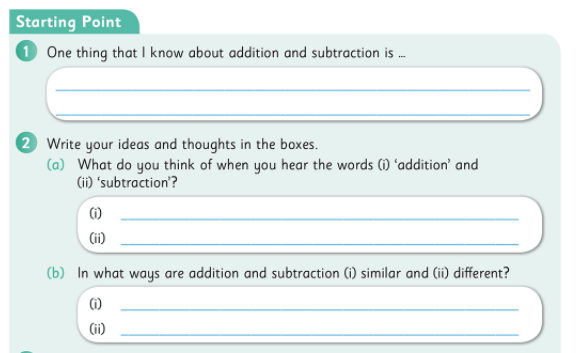In particular, the children are enabled to understand addition and subtraction as being the inverse of each other and are encouraged to use the inverse operation to check calculations.Looking at the bigger picture

Children can often have tunnel vision (or column vision) regarding addition and subtraction calculations: they “do” the units, then the tens, then the hundreds without really looking at the whole numbers or the processes involved.One way in which you can encourage the children to look at and understand these operations better is by using a Concrete–pictorial–abstract (CPA) approach. This means the children will be moving from experiences with the familiar base ten concrete materials (e.g. straws, base ten blocks, money, the Operation Maths place value discs, pictured above) to pictorial activities (e.g. where the children draw representations of the numbers using pictures of the concrete materials or use empty number lines, bar models, etc.) and finally to abstract exercises, where the focus is primarily on numbers and/or digits.

When exchanging tens and units or tens and hundreds, reinforce that a ten is also the same as 10 units, and that a hundred is the same as 10 tens and 100 units.
The use of non-canonical arrangements of numbers (e.g. representing 245 as 2H 3T 15U or 1H 14T 5U), as mentioned in Place Value, can also be very useful to children as they develop their ability to visualise the regrouping/renaming process. The Operation Maths Place Value eManipulative, accessible on edcolearning.ie,  is an excellent way to illustrate this and explore the operations in a visual way. Worth noting also, is that the Operation Maths Place Value eManipulative and place value discs provide the only means to concretely or pictorially represent base ten materials to five whole number places (no other interactive tool is available on the internet to do this); a fact which will be of particular value to teachers of 5th and 6th classes who didn’t have a way to concretely/visually represent numbers to ten thousands prior to the inception of Operation Maths.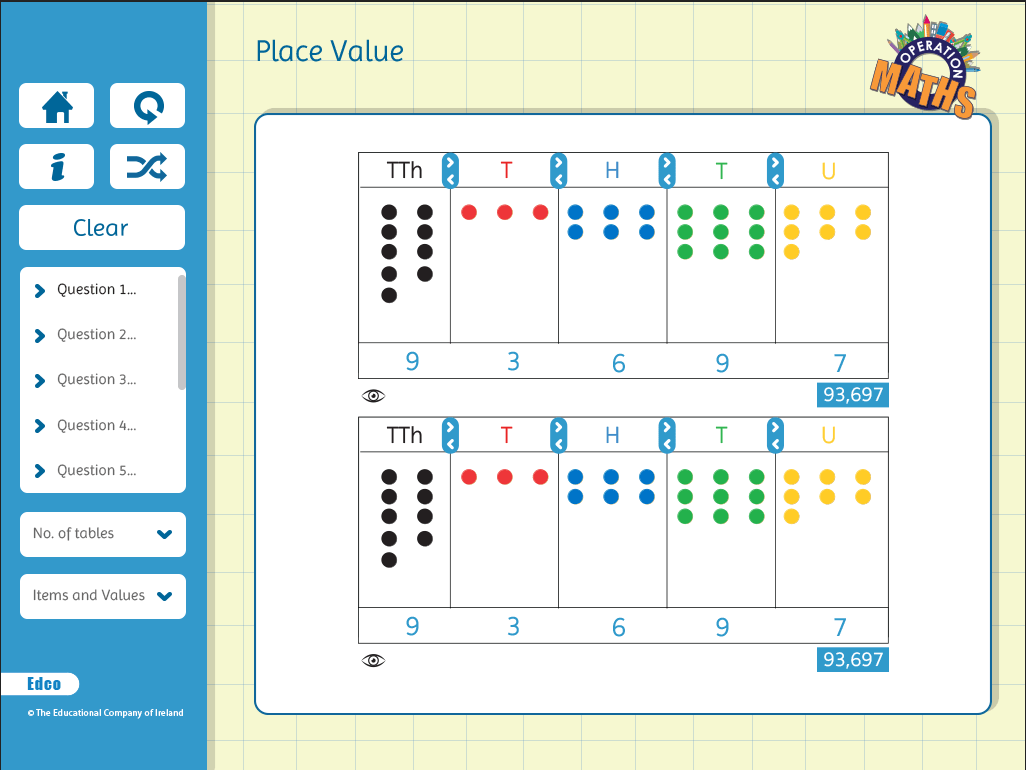Mental strategies are as important as written methods

The traditional, written algorithms for addition and subtraction, i.e. the column methods, are important aspects of these operations. However, in real-life maths, mental calculations are often more relevant than written methods. Also, as mentioned previously, children can often have tunnel vision (or column vision) regarding addition and subtraction calculations; they ‘do’ the units, then the tens, then the hundreds, etc., without really looking at the entire numbers or the processes involved. Therefore, while the column method for addition and subtraction is a main part of this topic, equally important is the development of mental calculation skills, using such strategies as those outlined on this page from Operation Maths 6 (below)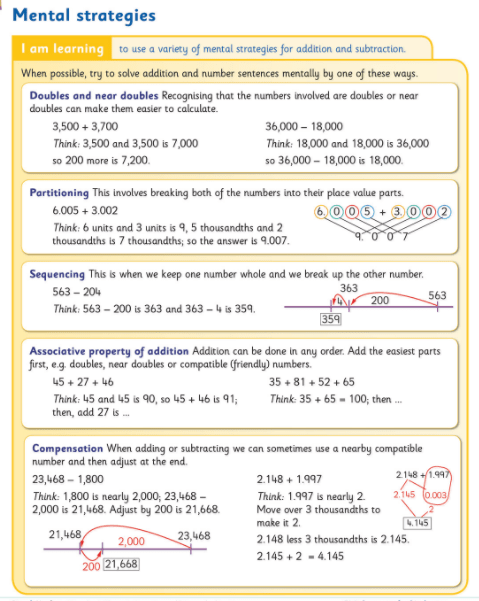Thus, one of the main purposes of the Addition and Subtraction chapters in Operation Maths is to extend the range of mental calculation strategies the children have and to enable them to apply the strategies to numbers of greater complexity i.e. for the children to become efficient and flexible, as well as accurate. As the same calculation can often be done mentally in many different ways, the children have to develop their decision-making skills so as to be in a position to decide what is the most efficient strategy to use in each situation.

It is worth noting that the page from Operation Maths 6 pictured above serves as a synopsis to remind the children of all the strategies they explored individually in the previous Operation Maths books. That said, if the sixth class children are new to Operation Maths and have never encountered these strategies before, they may need to progress at a much slower pace than those who have been using the programme previously, or who may have encountered these strategies, for example a class who used Number Talks. As mentioned in a previous post, the Operation Maths mental strategies listed below are very similar to, and in some cases identical to, those used in Number Talks (if different terminology from Operation Maths is used in Number Talks, the Number Talks terminology is given in brackets).

• Doubles and near doubles
• Number bonds of 10, 100 and 1,000 (Making tens)
• Friendly or Compatible numbers (benchmark/friendly numbers)
• Partitioning (breaking each number into its place value parts)
• Compensation
• Subtraction as take-away (removal/deducation)
• Constant difference subtraction (see below)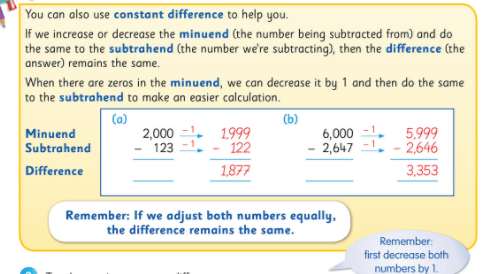Operation Maths also places particular emphasis on the development of estimation skills for number and introduces and develops specific estimation strategies as the books progress. Again, the emphasis is on the children contrasting and comparing these strategies and choosing the most efficient strategy each time. To find out more about some of the estimation strategies, read this post.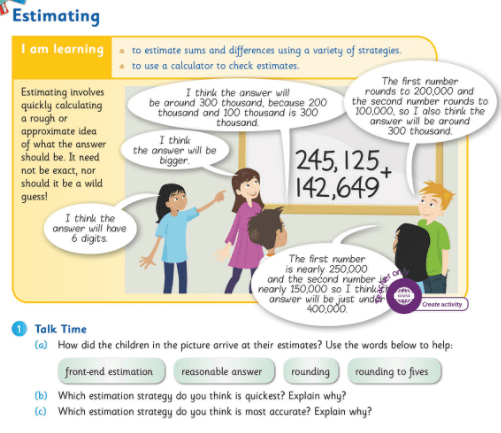Therefore, ask the children, as often as possible when meeting new calculations, can they do it mentally, and how, so that they become increasingly aware of a range of mental calculation skills and approaches. In this way the children will also be developing their decision-making skills, so as to be in a position to decide the most efficient strategy/approach to use.

Problem-solving strategies

One of the main aims of Operation Maths 3-6 was to introduce both teachers and pupils to a logical problem solving approach (i.e. RUCSAC) , complemented by specific visual problem solving strategies which develop in complexity as the child progresses through the senior classes.

A key step in the RUCSAC problem-solving approach is the ability to read a word problem meaningfully, and highlight the specific operational language or vocabulary. This is reinforced with activities in the Discovery Book (see below) where the children colour-code the specific phrases and then transfer them to their Operations Vocabulary page towards the end of their Discovery Book for future reference.You will notice that the problems have no numbers to distract the children, so that they can just focus on the language of the problems and the operations that may be inferred by the context of the story. These type of “numberless word problems” are being used more and more by practitioners in order to deepen children’s understanding of the concepts involved.

Another key step in the RUCSAC approach is the ability to create to show what you know, where the child makes a representation of the word problem in another form. Bar models are ideal for use with operational word problems. Introduced initially in Operation Maths 3, the use of bar models is developed through Operation Maths 3-6 to include bar models suited to other types of word problems.

Empty number lines can also be used to represent addition and subtraction problems (see below). In the senior books, the children will use both strategies to represent word problems and compare and contrast the two strategies. Ultimately, it is hoped that the children will use the strategy that they are most comfortable with. For more information on problem-solving strategies please consult the guide to problem-solving strategies across the scheme in the introduction to your Teachers Resource Book (TRB) or read on here.Communicating and expressing thinking

Being able to explain your mathematical thinking is a very powerful tool, and one that can greatly aid the learning and understanding of both the speaker and the listener(s). Encourage the children to verbalise how they did their calculations (mental or written) to provide you with a window on their thinking. When talking about decimal numbers, encourage children to use fractional language as opposed to decimal language, i.e. ‘6 hundredths plus 4 hundredths is ten hundredths’ etc.Another way to communicate and express thinking is via jottings. These are informal diagrams that both show and support thinking, and when used as a part-mental approach, serve as an intermediate stage between concrete materials and the abstract calculation. Their use should be encouraged as much as possible (e.g. “use jottings to show me your thinking”) until the child is confident enough to do the whole calculation mentally or using a traditional written form. The main jottings used in Operation Maths are empty number lines (pictured above) and branching (pictured below) to show part–whole relationships and/or explore compensation.• Dear Family, your Operation Maths Guide to Addition & Subtraction includes practical suggestions for supporting children, and links to a huge suite of digital resources, organised according to class level.
• Operation Maths Digital Resources: As always don’t forget to access the linked digital activities on the digital version of the Pupil’s book, available on edcolearning.ie. Tip: look at the footer on the first page of each chapter in the pupil’s book to get a synopsis of what digital resources are available/suggested to use with that particular chapter.
• Mental Maths handbook for Addition and Subtraction from the PDST
• Number Talks book by Sherry Parrish
• Addition & Subtraction Board on Pinterest
• This short video from Graham Fletcher showing the progression of addition and subtraction from the infant classes to the formal written algorithm, with three and four-digit numbers, is also worthwhile viewing:

Operation Maths: also the most child-friendly primary maths programme!Not only is Operation Maths the most teacher-friendly programme currently available, but it is also the most child-friendly. Read on to find out why.

Enabling children to understand maths, not just do maths

In case you haven’t picked up on it already, Operation Maths is all about understanding, understanding, understanding!

As mentioned before, Operation Maths is based on a concrete, pictorial, abstract (or CPA) approach.

Concrete activities and experiences are emphasised and outlined throughout the pupil books. The Teachers Resource Book (TRB) also provides further suggestion for the ways in which a teacher can use concrete activities with their class.
These experiences are then further developed via the pictorial activities; this may be through interaction with the digital resources and/or via the write-in activities in the books. Of the CPA approach, exploring pictorial representations of materials, numbers, shapes etc., is a vital (but sometimes neglected, particularly at the senior end) step on a child’s journey towards understanding.
All of this should greatly enhance the children’s ability to visualise and understand maths and be more prepared when they meet the more traditional, abstract activities.

Active participation

The high number of concrete based activities within Operation Maths ensures that the children have regular, if not daily, opportunities to be engaged in active learning. The inclusion of free mini-whiteboards  (MWBs) as part of the programme also encourages the active involvement of all the class in any activities as all children must visibly participate. Moreover, the custom-made suite of write-hide-show videos and Maths around us videos (see below for samples) further increase the opportunities to use the MWBs. I challenge you to show the estimation video to your class and not have them all excited and active!

Mastery curriculum

Research suggests that changing chapters/topics every week, especially in senior end and especially the case of number topics, is not conducive to developing a strong understanding for the various topics and that preferable to this is a mastery curriculum approach, where the children are afforded a longer time to engage with, and ultimately master, the concepts. Therefore, in Operation Maths, these topics have a dedicated two weeks block, allowing the children to fully engage with the concepts before moving to a different topic, thereby increasing the likelihood for the children to master the content. So, if you look at the contents page of the Operation Maths books, you will notice that there are fewer chapters than usual but that many of these are “double” chapters, designed to be taught over ten school days, as opposed to five.

A better understanding of the operations

Similarly, research suggests that teaching opposite or inverse operations together, allows the children to better grasp the connections between the two, therefore promoting deeper conceptual understanding. So, in the senior end, addition and subtraction are taught together as part of the same chapter(s), as are multiplication and division. A similar approach is taken to the operations in first and second class once the children have been formally introduced to subtraction.

A seamless transition…

I was primarily involved in authoring all of the number and algebra chapters in the 3rd to 6th class books, while my co-authors Michael Browne and Siobhán Kelleher authored the chapters for measures, data, shape and space for the same classes. This means that, for the children, their experience of the content is very cohesive as it flows seamlessly from one class to the next.
Similarly the 5th and 6th class books were written in light of the NCCA Bridging documents and Project Maths at junior cycle in second level. In particular, extra attention was paid to the correct and accurate use of mathematical terminology which also helps ensure that the child’s transition from primary maths to second level maths is also as seamless as possible.

Other ways in which Operation Maths promotes the development of deeper connections and understanding include:

• Linkage: the topics are all taught in a very connected way, with cross references being made where appropriate. In particular, measures are not just confined to their own chapters: they are taught in an integrated way across all suitable chapters and particularly across number• Integration: The use of maths across other subjects is also emphasised regularly and particularly as part of the suggested activities in the TRBs. In the senior end there are also themed revision pages in the Pupil’s Book, where maths is explored through History, Geography, Sports etc.
• The books have a clean, uncluttered design making them easier for children to focus on the concepts and not be distracted by unnecessary images
• In the senior end, the Pupil’s Book also has clear and detailed teaching panels (easily identifiable by their yellow background) right throughout each chapter, to aid both the teachers and the children, and can be particularly useful if the children are working independently or in small groups.
• To help make connections with the environment there are dedicated Maths Around Us videos in the digital resources, Maths Around Us activities in the Pupil’s Book and Maths Trails in the TRB photocopiables and in selected Discovery Books